# Radial Basis Functions for Computer Graphics Contents 1

• Slides: 40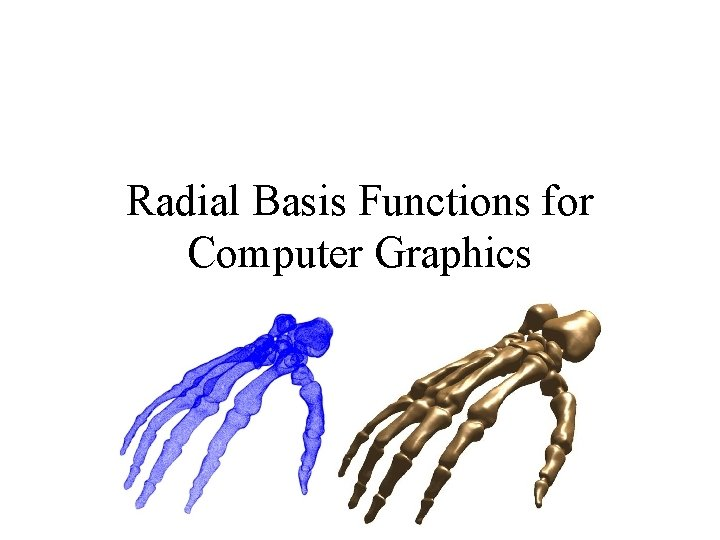Radial Basis Functions for Computer Graphics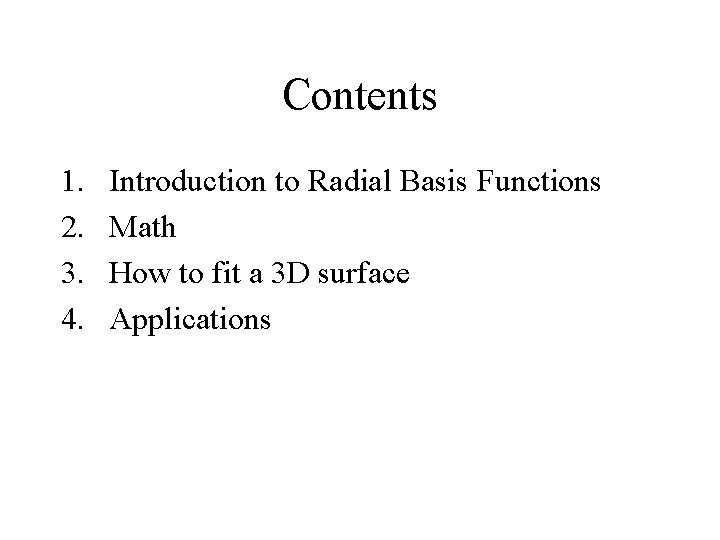Contents 1. 2. 3. 4. Introduction to Radial Basis Functions Math How to fit a 3 D surface ApplicationsWhat can Radial Basis Functions do for me? (A short introduction)An RBF takes these points:And gives you this surface: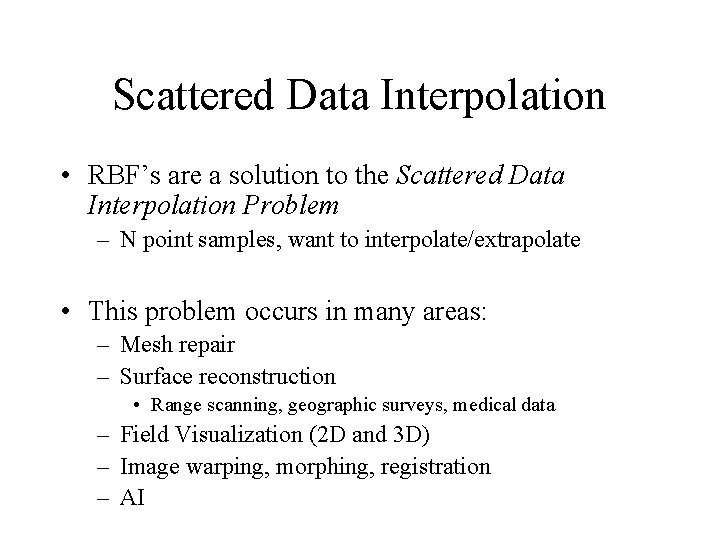Scattered Data Interpolation • RBF’s are a solution to the Scattered Data Interpolation Problem – N point samples, want to interpolate/extrapolate • This problem occurs in many areas: – Mesh repair – Surface reconstruction • Range scanning, geographic surveys, medical data – Field Visualization (2 D and 3 D) – Image warping, morphing, registration – AIHistory Lesson • Discovered by Duchon in 77 • Applications to Graphics: – Savchenko, Pasko, Okunev, Kunii – 1995 • Basic RBF, complicated topology bits – Turk & O’Brien – 1999 • ‘variational implicit surfaces’ • Interactive modeling, shape transformation – Carr et al • 1997 – Medical Imaging • 2001 – Fast Reconstruction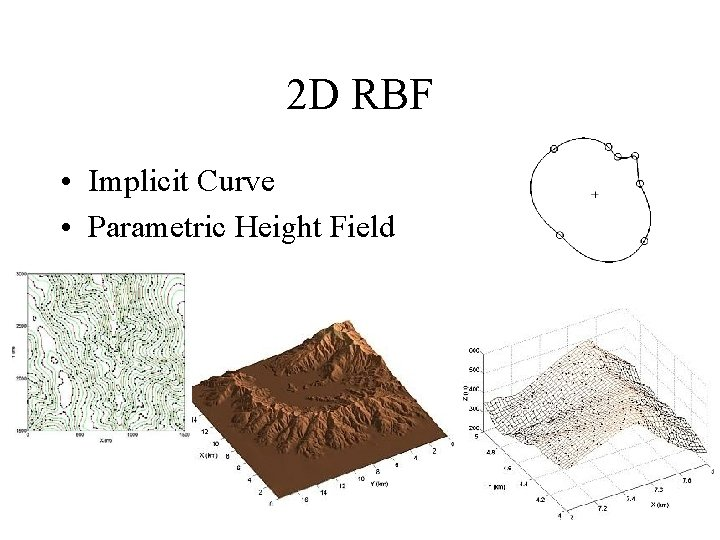2 D RBF • Implicit Curve • Parametric Height Field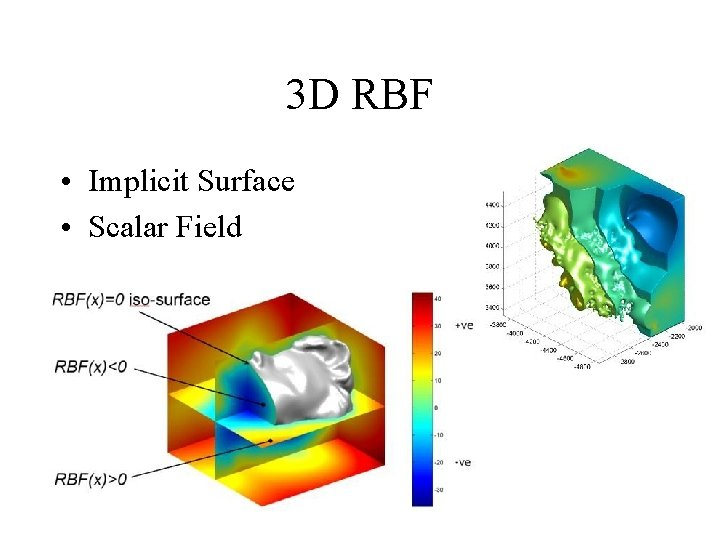3 D RBF • Implicit Surface • Scalar Field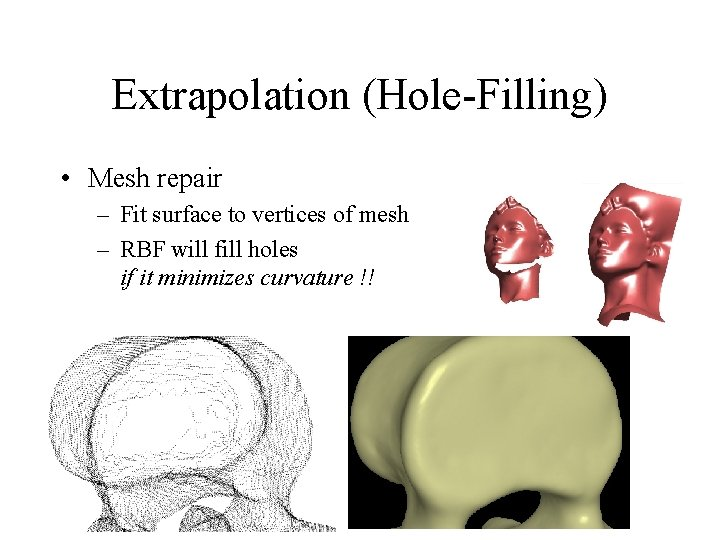Extrapolation (Hole-Filling) • Mesh repair – Fit surface to vertices of mesh – RBF will fill holes if it minimizes curvature !!Smoothing • Smooth out noisy range scan data • Repair my rough segmentation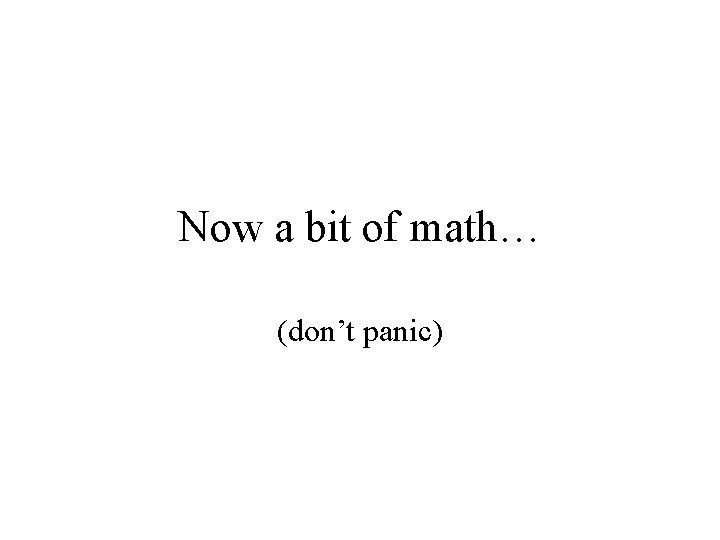Now a bit of math… (don’t panic)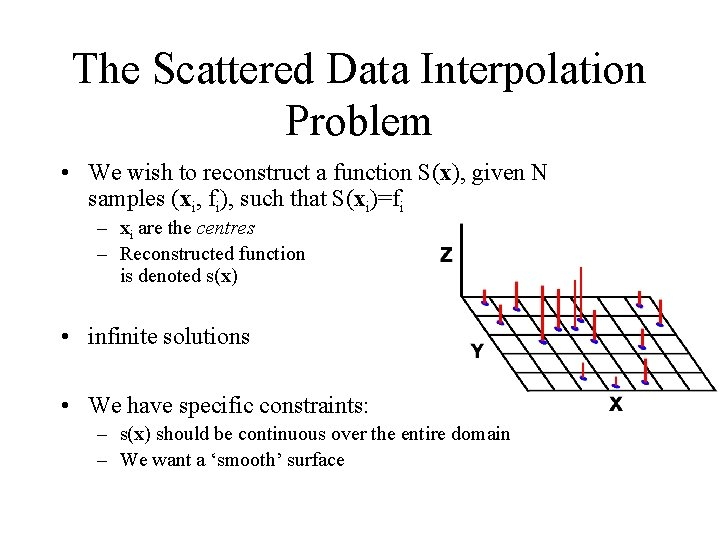The Scattered Data Interpolation Problem • We wish to reconstruct a function S(x), given N samples (xi, fi), such that S(xi)=fi – xi are the centres – Reconstructed function is denoted s(x) • infinite solutions • We have specific constraints: – s(x) should be continuous over the entire domain – We want a ‘smooth’ surface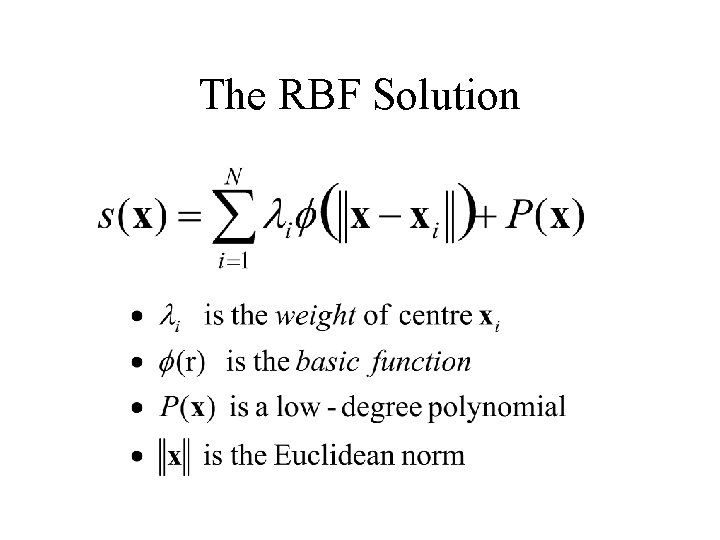The RBF Solution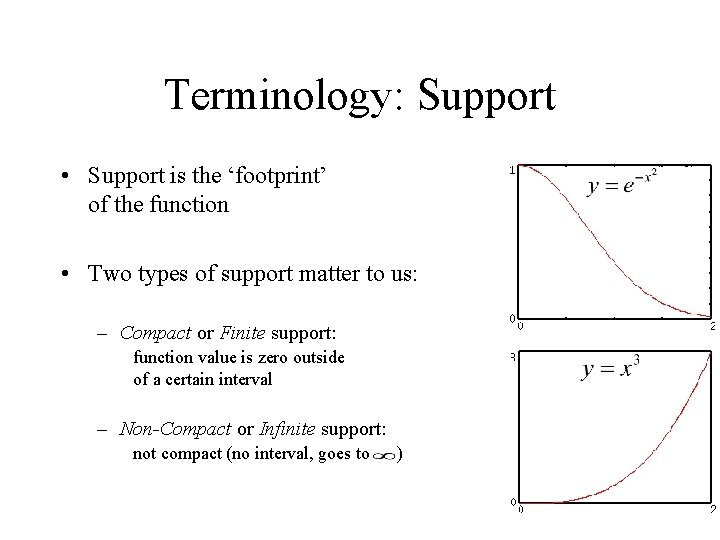Terminology: Support • Support is the ‘footprint’ of the function • Two types of support matter to us: – Compact or Finite support: function value is zero outside of a certain interval – Non-Compact or Infinite support: not compact (no interval, goes to )Basic Functions ( ) • Can be any function – Difficult to define properties of the RBF for an arbitrary basic function • Support of function has major implications – A non-compactly supported basic function implies a global solution, dependent on all centres! • allows extrapolation (hole-filling)Standard Basic Functions • Polyharmonics (Cn continuity) – 2 D: – 3 D: • Multiquadric: • Gaussian: – compact support, used in AI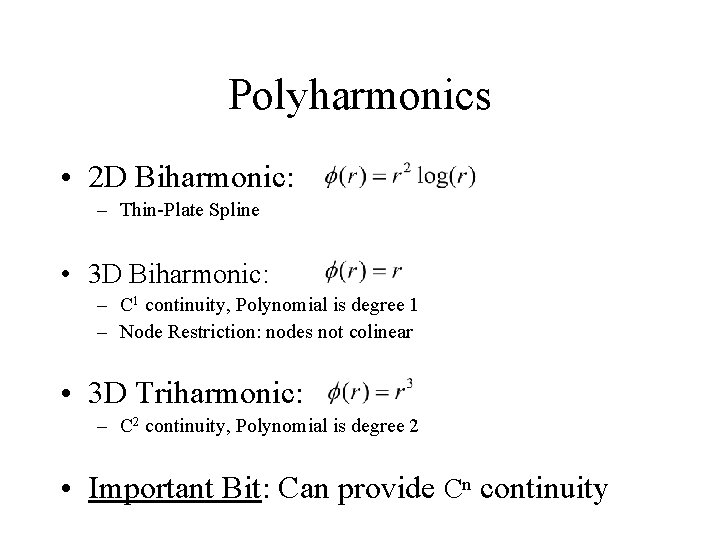Polyharmonics • 2 D Biharmonic: – Thin-Plate Spline • 3 D Biharmonic: – C 1 continuity, Polynomial is degree 1 – Node Restriction: nodes not colinear • 3 D Triharmonic: – C 2 continuity, Polynomial is degree 2 • Important Bit: Can provide Cn continuity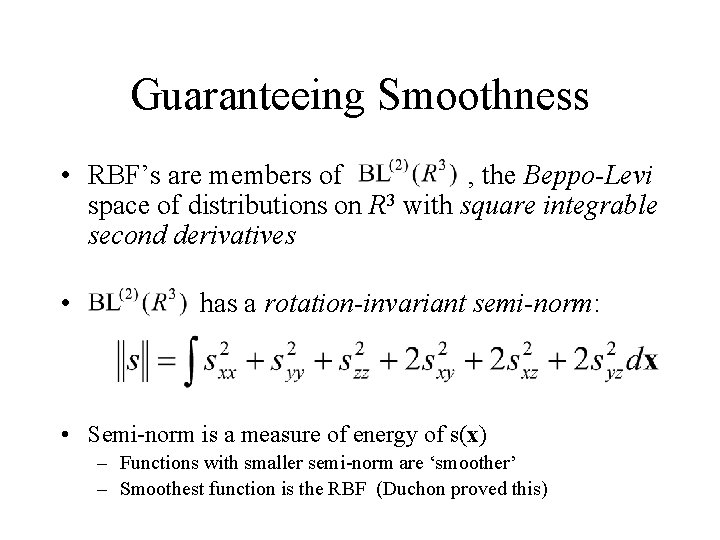Guaranteeing Smoothness • RBF’s are members of , the Beppo-Levi space of distributions on R 3 with square integrable second derivatives • has a rotation-invariant semi-norm: • Semi-norm is a measure of energy of s(x) – Functions with smaller semi-norm are ‘smoother’ – Smoothest function is the RBF (Duchon proved this)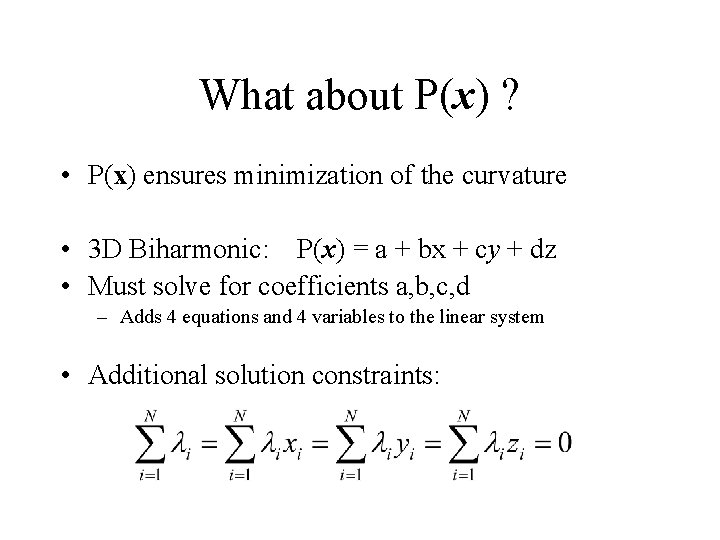What about P(x) ? • P(x) ensures minimization of the curvature • 3 D Biharmonic: P(x) = a + bx + cy + dz • Must solve for coefficients a, b, c, d – Adds 4 equations and 4 variables to the linear system • Additional solution constraints:Finding an RBF Solution • The weights and polynomial coefficients are unknowns • We know N values of s(x): • We also have 4 side conditions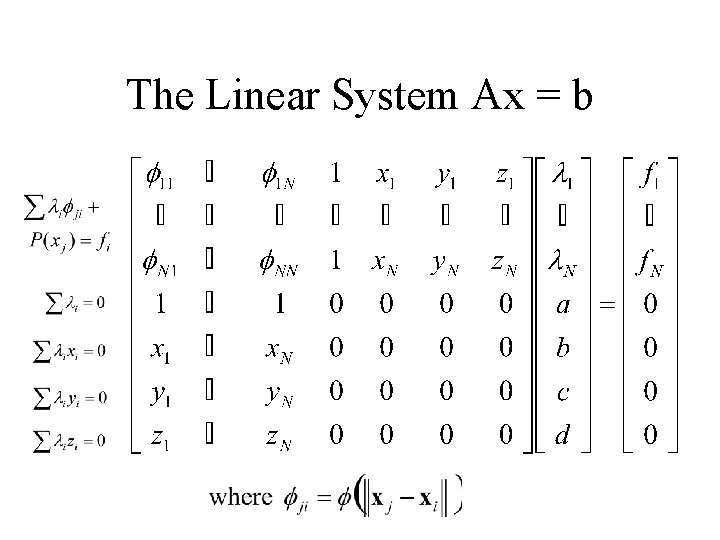The Linear System Ax = b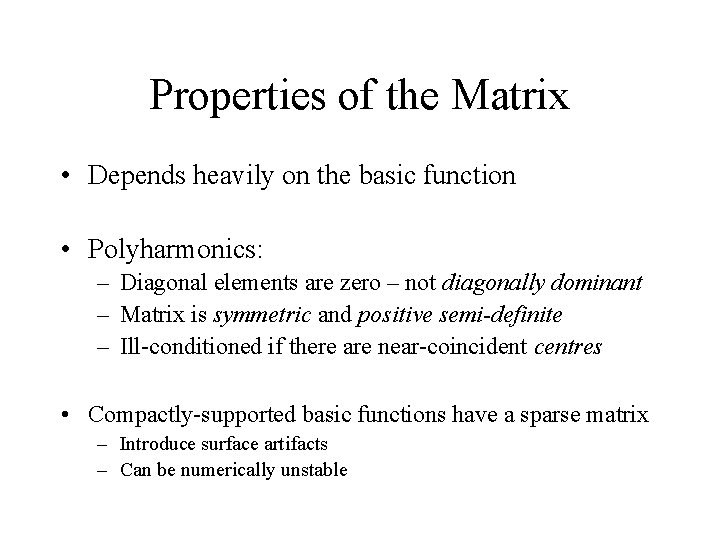Properties of the Matrix • Depends heavily on the basic function • Polyharmonics: – Diagonal elements are zero – not diagonally dominant – Matrix is symmetric and positive semi-definite – Ill-conditioned if there are near-coincident centres • Compactly-supported basic functions have a sparse matrix – Introduce surface artifacts – Can be numerically unstable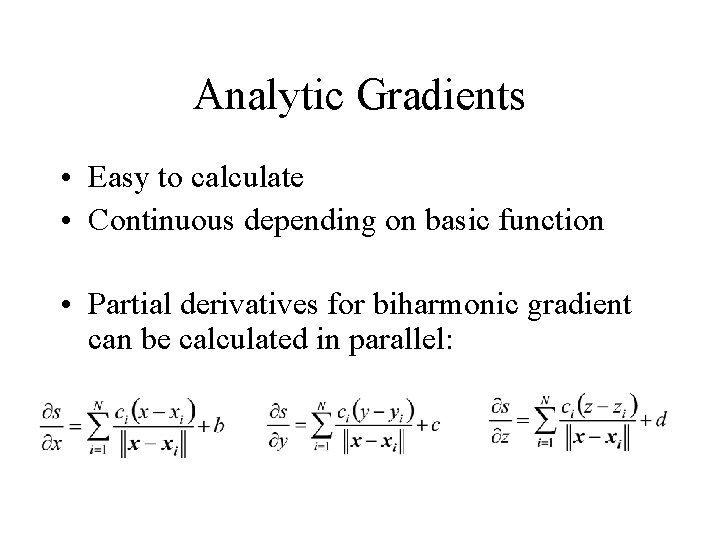Analytic Gradients • Easy to calculate • Continuous depending on basic function • Partial derivatives for biharmonic gradient can be calculated in parallel: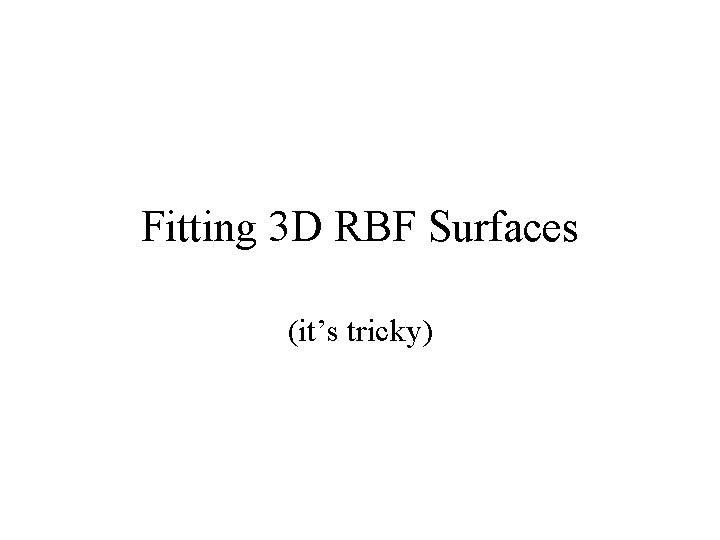Fitting 3 D RBF Surfaces (it’s tricky)Basic Procedure 1. 2. Acquire N surface points Assign them all the value 0 (This will be the iso-value for the surface) 3. Solve the system, polygonize, and render:Off-Surface Points • Why did we get a blank screen? – Matrix was Ax = 0 – Trivial solution is s(x) = 0 – We need to constrain the system • Solution: Off-Surface Points – Points inside and outside of surface • Project new centres along point normals • Assign values: <0 inside; >0 outside • Projection distance has a large effect on smoothnessInvalid Off-Surface Points • Have to make sure that off-surface points stay inside/outside surface! – Nearest-Neighbor test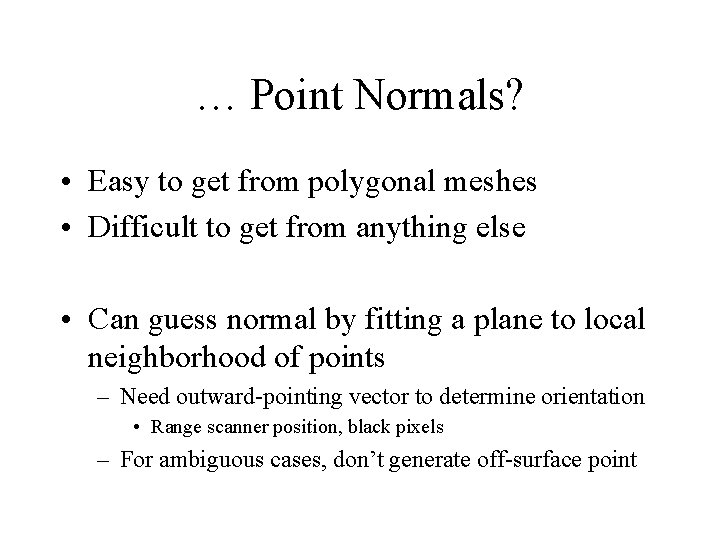… Point Normals? • Easy to get from polygonal meshes • Difficult to get from anything else • Can guess normal by fitting a plane to local neighborhood of points – Need outward-pointing vector to determine orientation • Range scanner position, black pixels – For ambiguous cases, don’t generate off-surface point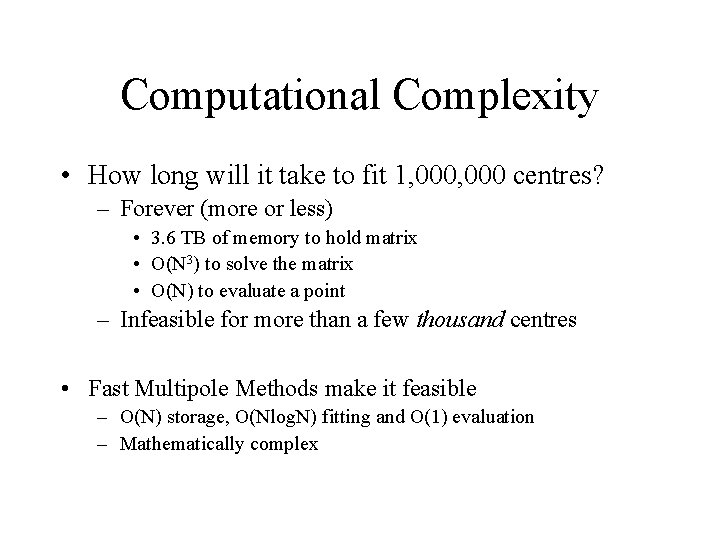Computational Complexity • How long will it take to fit 1, 000 centres? – Forever (more or less) • 3. 6 TB of memory to hold matrix • O(N 3) to solve the matrix • O(N) to evaluate a point – Infeasible for more than a few thousand centres • Fast Multipole Methods make it feasible – O(N) storage, O(Nlog. N) fitting and O(1) evaluation – Mathematically complex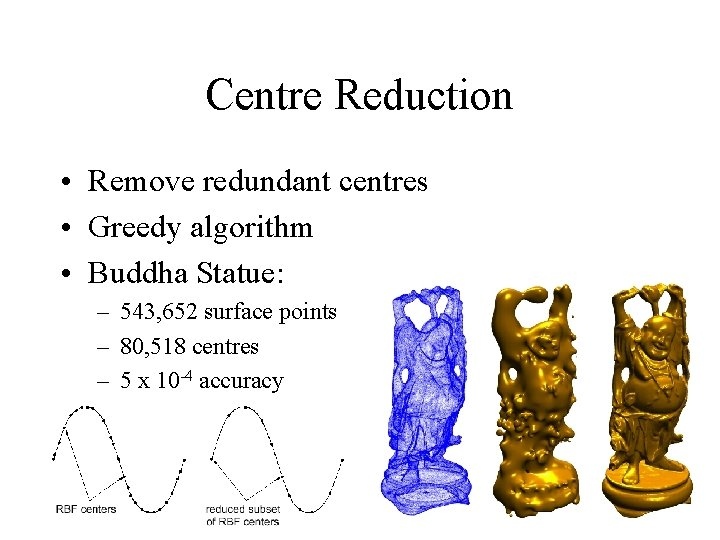Centre Reduction • Remove redundant centres • Greedy algorithm • Buddha Statue: – 543, 652 surface points – 80, 518 centres – 5 x 10 -4 accuracyFast. RBF • Far. Field. Technology (. com) • Commercial implementation – 3 D biharmonic fitter with Fast Multipole Methods – Adaptive Polygonizer that generates optimized triangles – Grid and Point-Set evaluation • Expensive – They have a free demo limited to 30 k centres • Use iterative reduction to fit surfaces with more pointsApplications (and eye candy)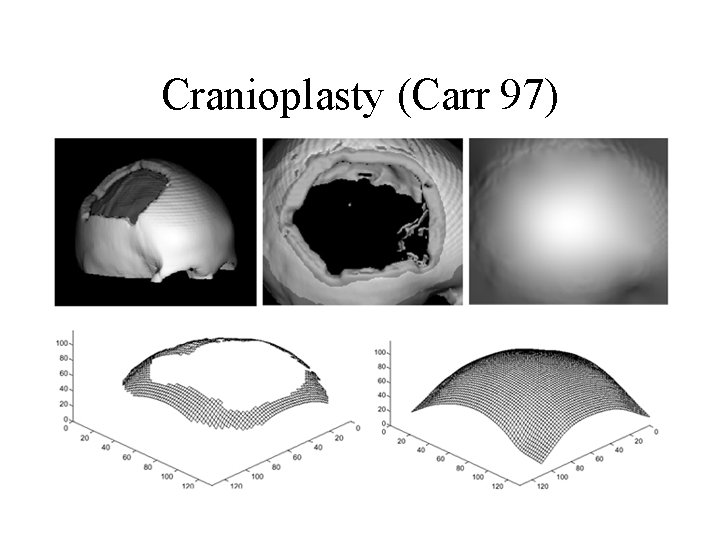Cranioplasty (Carr 97)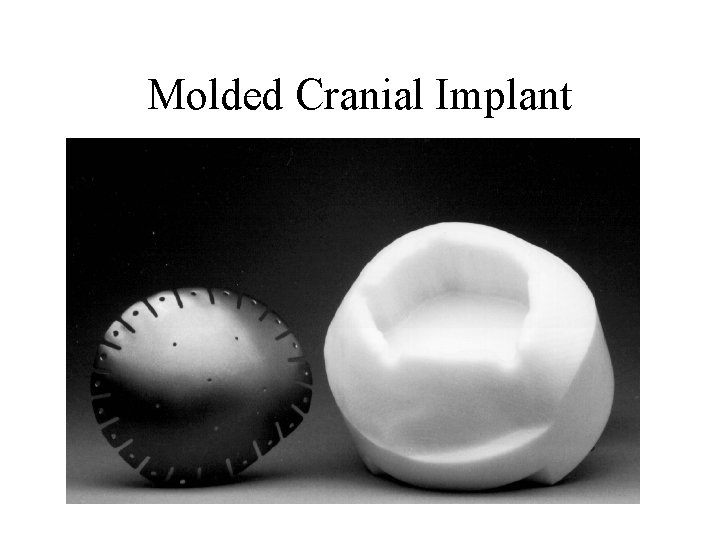Molded Cranial Implant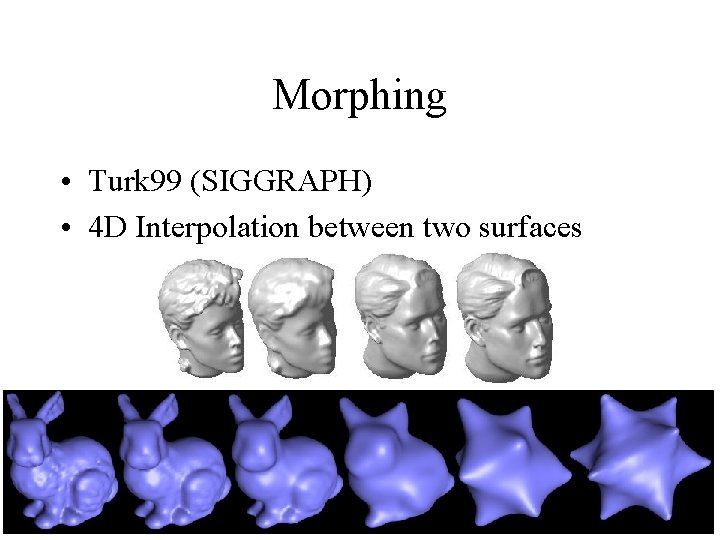Morphing • Turk 99 (SIGGRAPH) • 4 D Interpolation between two surfacesMorphing With Influence Shapes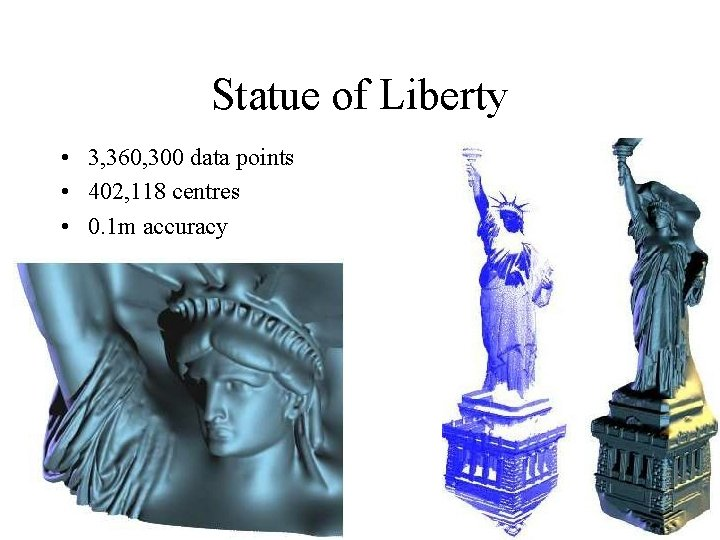Statue of Liberty • 3, 360, 300 data points • 402, 118 centres • 0. 1 m accuracy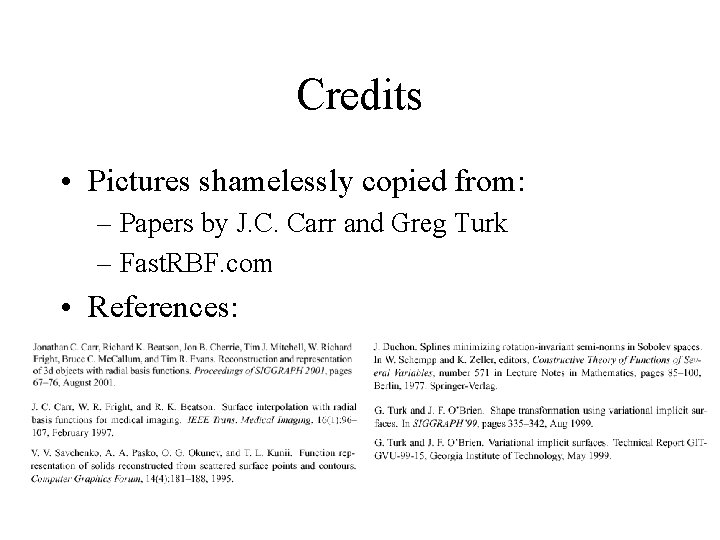Credits • Pictures shamelessly copied from: – Papers by J. C. Carr and Greg Turk – Fast. RBF. com • References: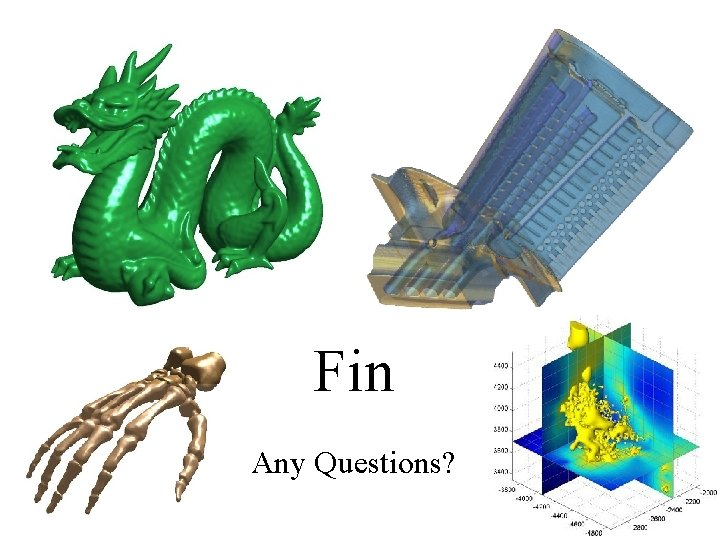Fin Any Questions?